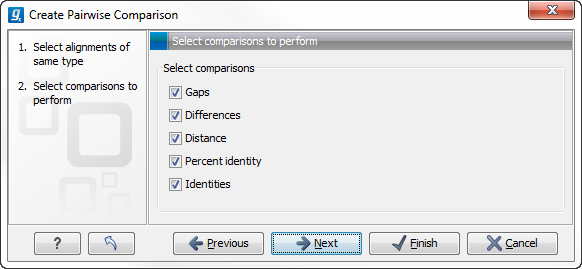## Pairwise comparison parameters

There are five kinds of comparison that can be made between the sequences in the alignment, as shown in figure 13.17.Figure 13.17: Adjusting parameters for pairwise comparison.

• Gaps Calculates the number of alignment positions where one sequence has a gap and the other does not.
• Identities Calculates the number of identical alignment positions to overlapping alignment positions between the two sequences. An overlapping alignment position is a position where at least one residue is present, rather than only gaps.
• Differences Calculates the number of alignment positions where one sequence is different from the other. This includes gap differences as in the Gaps comparison.
• Distance Calculates the Jukes-Cantor distance between the two sequences. This number is given as the Jukes-Cantor correction of the proportion between identical and overlapping alignment positions between the two sequences.
• Percent identity Calculates the percentage of identical residues in alignment positions to overlapping alignment positions between the two sequences.